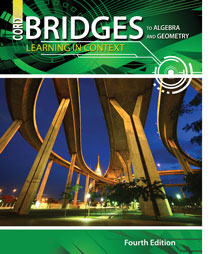# Bridges to Algebra and Geometry - 4th Edition

## Chapter 6: Ratio, Proportion, and Probability

Some links are repeated for use with more than one lesson.

### 6.1 Ratio and Proportion

http://www.mathsisfun.com/numbers/ratio.html
NEW This page presents several examples of working with ratios and includes some practice problems.

http://www.mathsisfun.com/algebra/cross-multiply.html
NEW This page shows how cross-multiplying works to check for equal ratios.

### 6.2 Rates and Conversions

http://www.shodor.org/unchem-old/math/units/index.html
NEW This page gives a detailed discussion of the important role played by units of measure, how to simplify units, and how to use unit ratios to convert units of measure.

http://www.convert-me.com/en/
NEW This site provides an exhaustive list of conversion factors for all types of measures, with which you can form unit ratios for your measurement calculations.

### 6.3 Using Proportion

http://www.math.com/school/subject1/lessons/S1U2L2GL.html
This site defines ratio and shows examples.

http://www.explorelearning.com/index.cfm?method=cResource.dspResourcesForCourse&CourseID=213
This site has examples and problems for the students to solve that involve ratios, proportions, and percents.

### 6.4 Sampling

http://www.nationmaster.com/encyclopedia/Sampling-(statistics)
This site defines sampling.

http://pages.csam.montclair.edu/~mcdougal/SCP/Sampling.htm
NEW This page presents a discussion of different sampling strategies, and explanations why some would be considered biased.

### 6.5 Probability

http://www.cut-the-knot.org/probability.shtml
This site discusses probability and gives multiple problems for the students to solve.

http://www.mathsisfun.com/data/probability.html
NEW This page presents good vocabulary and definitions for probability problems, and some practice probability problems.

### 6.6 Sample Space

http://www.algebra-class.com/fundamental-counting-principle.html
NEW This page presents several examples using the fundamental counting principle to determine sample space.

http://www.mathsisfun.com/data/probability-tree-diagrams.html
NEW This page has a nice presentation of using tree diagrams to help determine a sample space and probabilities

### 6.7 Independent and Dependent Events

http://www.mathsisfun.com/data/probability-events-independent.html
NEW This page has a nice explanation and several examples of events, with some practice problems.

http://www.regentsprep.org/Regents/math/ALGEBRA/APR6/Lindep.htm
NEW This site has a concise definition and examples of independent and dependent events.

### 6.8 Experimental Probability and Simulation

http://www.regentsprep.org/Regents/math/ALGEBRA/APR5/theoProp.htm
NEW This site compares experimental (or empirical) probability with theoretical probability.‎

http://www.shodor.org/interactivate/activities/ExpProbability/
NEW This site includes an interactive simulator that tallies the results of spinners and dice.